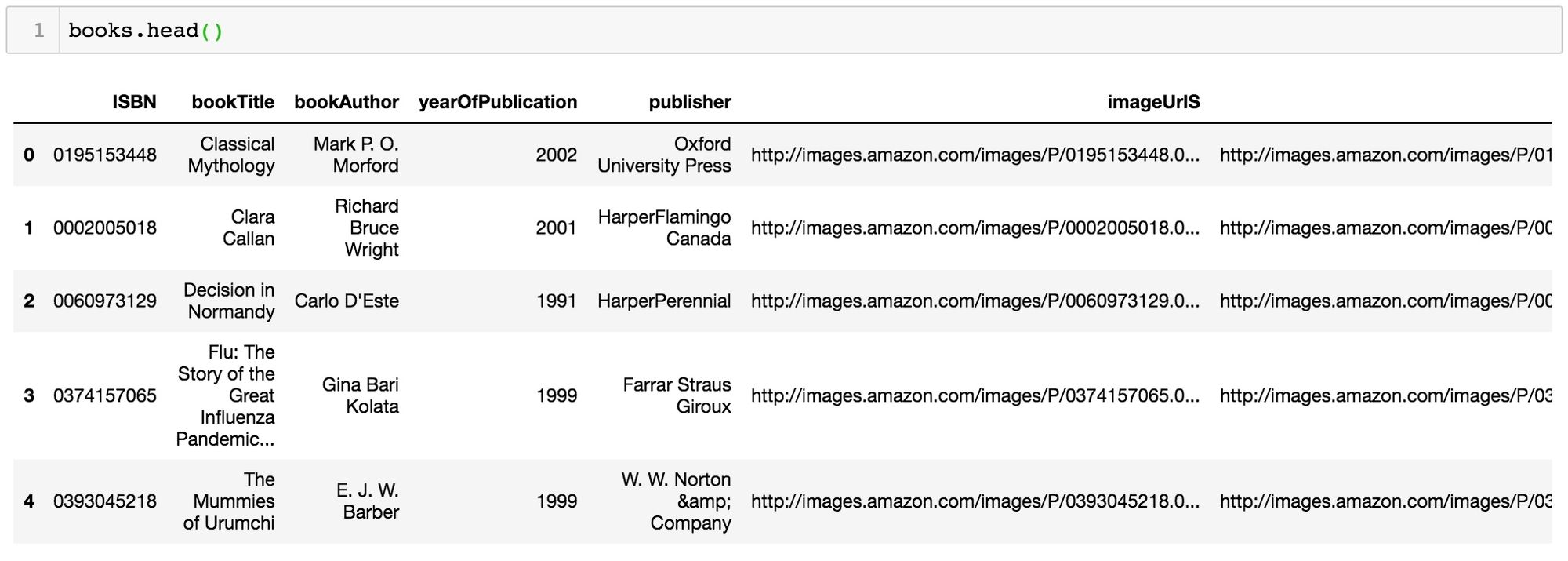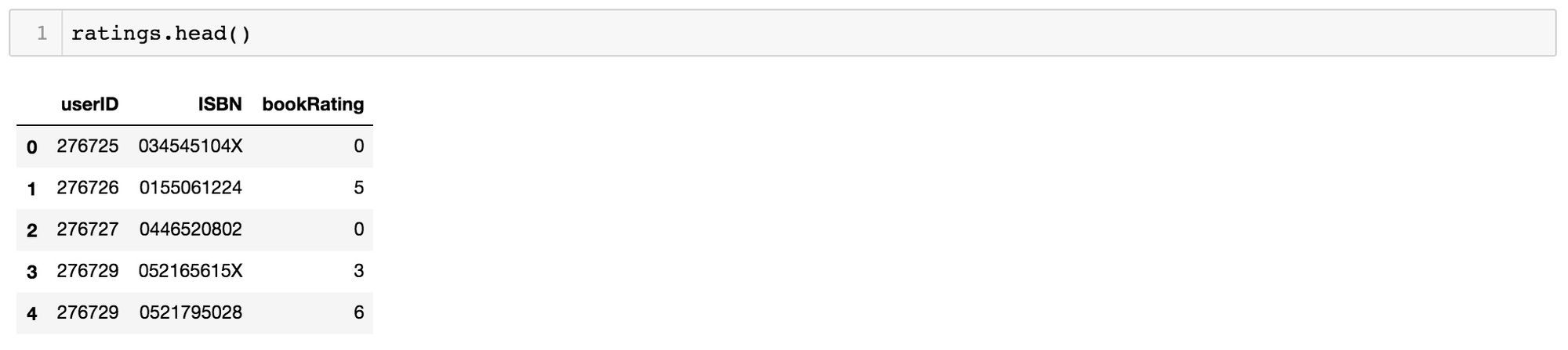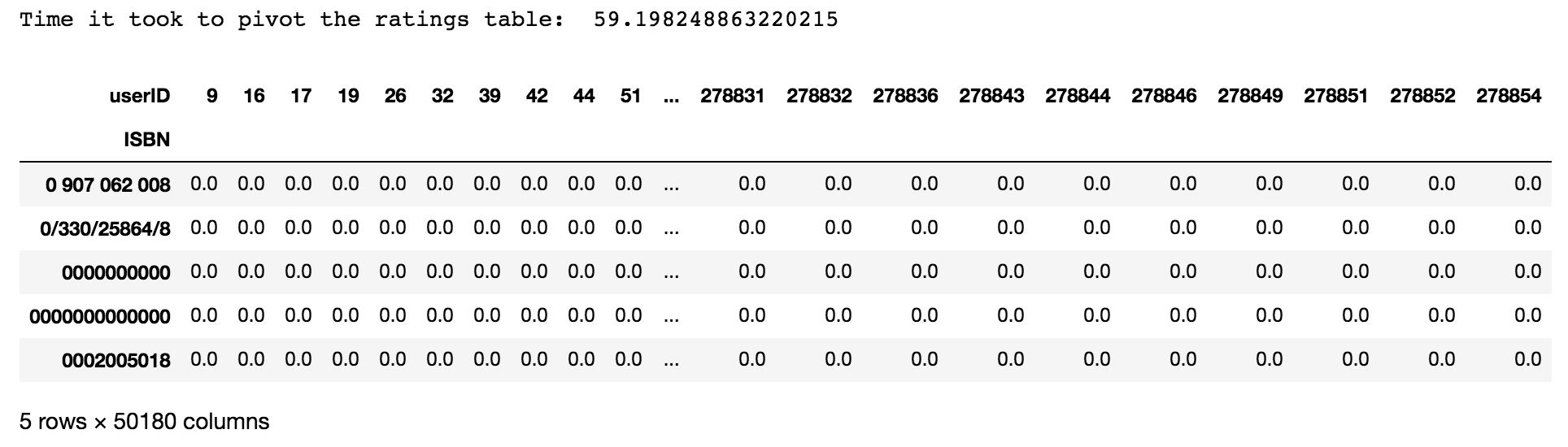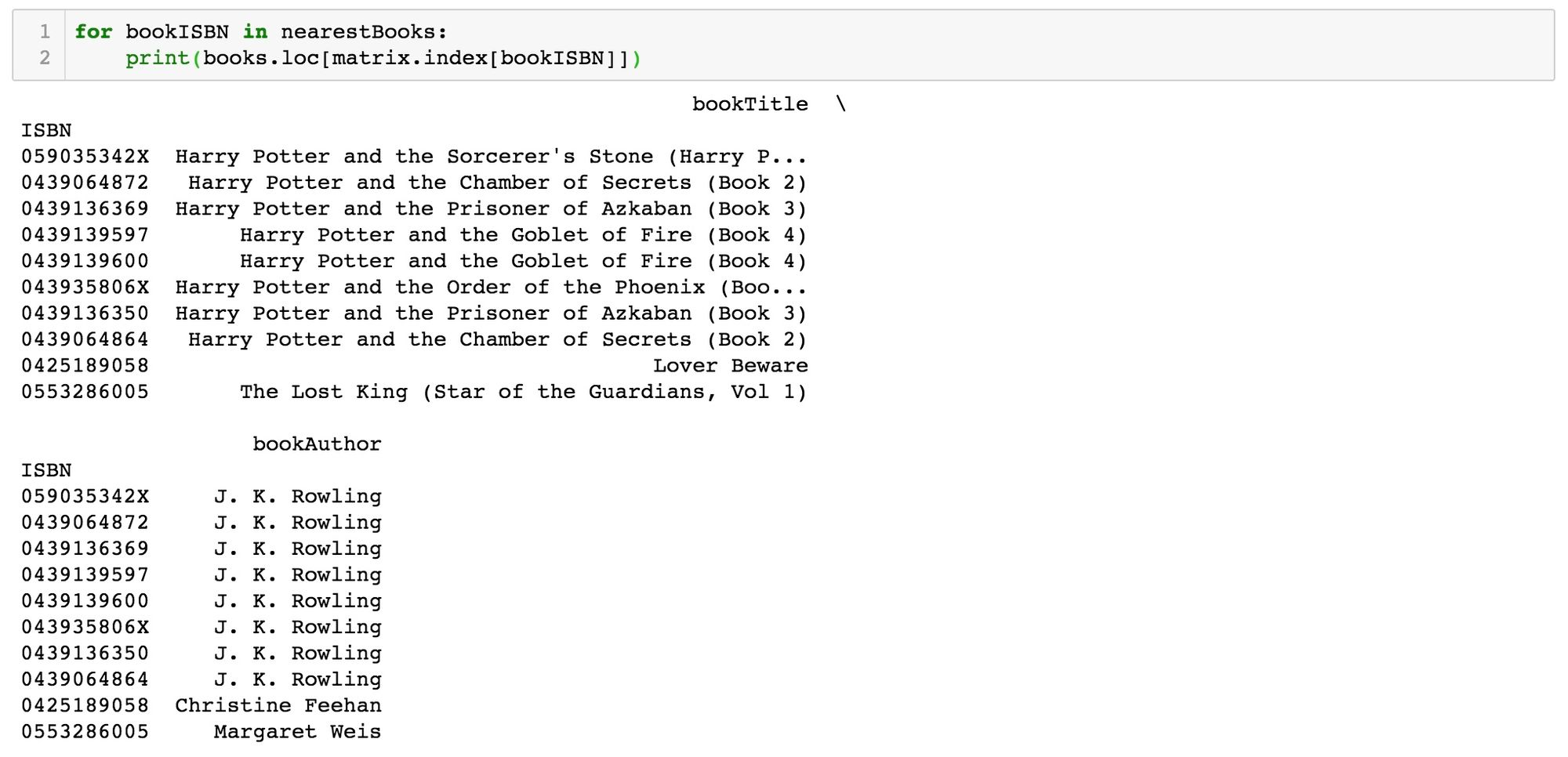# CPU or GPU for your recommendation engine?

Deploy
Olga Petrova

In today's data-driven world, GPUs are the hardware of choice for training Deep Learning models. What about tasks that do not involve artificial neural networks? For instance, is there a benefit to using a GPU for making product recommendations? Continue reading to find out!

Anyone selling anything these days makes recommendations. "Customers who bought this item also bought these ones." "Here are the top 10 TV series that we bet you'll enjoy." Sometimes these recommendations are based on the intrinsic properties of the products, but more often they come from the behaviours of users such as yourself.Let us say we want to build a simple book recommender system. The data that we need for it is available on any website containing users' reviews of books: e.g. this dataset has been collected from BookCrossing.com, a website dedicated to the practice of "releasing books into the wild" - leaving them in public places to be picked up and read by other members of the community. There are three data tables available, but we will only be needing two of them today: `BX-Books` and `BX-Book-Ratings` containing information on the books and the bookcrossers' book ratings respectively (pardon the excessive use of book in the preceding sentence, finding a suitable synonym is no easy task!). Each book in `BX-Books` is identified by a unique ISBN, and each row of `BX-Book-Ratings` lists the ISBN of the title that the user's rating refers to.## Using cosine similarity to make product recommendations

First, let us discuss how the ratings can be leveraged to generate appropriate book recommendations. If you are already familiar with the basics of recommender systems (or simply uninterested in the details), feel free to skip to the next section for the CPU vs. GPU comparison.

For each book, there are multiple ratings posted by different users, and it is this information that we will be using to infer the "likeliness" of the books from. Consider Harry Potter and the Sorcerer's Stone, Harry Potter and the Chamber of Secrets (the first two tomes of the series), and a textbook called Quantum Computation and Quantum Information. To illustrate with a simple example, let us say we have a total of five readers. Four of them read both Harry Potter books and ranked them highly, and one of the four has also enjoyed reading about quantum information:

HP 1
7
8
7
9
8
HP 2
8
8
9
6
-
QCQI
10
-
-
-
-

Eric (that's what E stands for) has made a New Year's resolution to read more in 2020, and, as it often happens, made no effort in keeping to it until mid year. Eric read the first Harry Potter book, and would like to use the book ratings of his friends to decide what to read next. How can he do that?

First, let's replace the `-` (not read) signs in the table above with zeros:

HP1
7
8
7
9
8
HP2
8
8
9
6
0
QCQI
10
0
0
0
0

Now, each book corresponds to a 5-dimensional vector containing the scores each reader has assigned to it. Eric would like to know which book, Harry Potter 2 or the quantum information textbook, would be most similar to Harry Potter 1 in terms of the readers' feedback. Mathematically this means that we are going to consider two pairs of vectors: HP1 and HP2, and HP1 and QCQI. A popular measure of how similar two vectors are, is called the cosine similarity, given by the dot product of the two vectors divided by the product of their magnitudes:

cos(θ) = A·B / ||A|| ||B||

When two vectors are aligned with one another, the cosine of the (zero) angle between them is 1, meaning the similarity is maximised. The similarity is zero for two vectors that are perpendicular to each other (e.g. if there is no overlap in the users who read the two books), and can also be negative if we allowed for negative ratings in our data table. The cosines for the two pairs in question are calculated as follows:

COS(Θ)
HP1 & HP2
(7x8+8x8+7x9+9x6) / [Sqrt(72+82+72+92+82) Sqrt(82+82+92+62)]
0.86
HP1 & QCQI
(7x10) / [Sqrt(72+82+72+92+82) Sqrt(102)]
0.40

Thus, when we make a recommendation on which book to read next based on Eric's interest in Harry Potter and the Sorcerer's Stone, Harry Potter and the Chamber of Secrets is a good bet (much better than Quantum Computation and Quantum Information!)

As you can imagine, the amount of effort to calculate cosine similarities for each pair of vectors grows quite quickly with the number of books as well as the number of users. Let us first see how long this will take on a CPU (10 core vCPU from a high end Intel Xeon Gold 6148 processor to be precise), using the popular `pandas` and `sklearn` libraries.

## The CPU route

Let us start by loading the data using `pandas`.

``import pandas as pddatadir = 'reco/BX-CSV-Dump/'books = pd.read_csv(datadir+'BX-Books.csv', sep=';', error_bad_lines=False, encoding="latin-1")books.columns = ['ISBN', 'bookTitle', 'bookAuthor', 'yearOfPublication', 'publisher', 'imageUrlS', 'imageUrlM', 'imageUrlL']ratings = pd.read_csv(datadir+'BX-Book-Ratings.csv', sep=';', error_bad_lines=False, encoding="latin-1")ratings.columns = ['userID', 'ISBN', 'bookRating']``

Now we can inspect the contents of the two tables:andUnless your final goal is to be able to say: "Here is the list of books that users with reading history similar to yours may or may not have hated with passion", you will probably want to remove all ratings below a certain threshold:

``# Keep only Ratings above 5:ratings = ratings[ratings.bookRating > 5]``

I will also drop the columns that we will not be needing from the Books table, make sure each ISBN in it corresponds to a single book entry, and set ISBN as the table's index:

``columns = ['yearOfPublication', 'publisher', 'imageUrlS', 'imageUrlM', 'imageUrlL']books = books.drop(columns, axis=1)books = books.drop_duplicates(subset='ISBN', keep="first")books = books.set_index('ISBN', verify_integrity=True)``

An additional pre-processing step that we will take is to filter out books that have been rated by fewer than three users. This filter will be applied to the Ratings, rather than the Books table:

``# Keep only those books, that have at least 2 ratings:ratings_count = ratings.groupby(by='ISBN')['bookRating'].count().reset_index().rename(columns={'bookRating':'ratingCount'})ratings = pd.merge(ratings, ratings_count, on='ISBN')ratings = ratings[ratings.ratingCount > 2]ratings = ratings.drop(['ratingCount'], axis=1)print(ratings.shape)``

This leaves us with 207,073 book ratings. Now let's get them into the form that we can use to compute the cosine similarities as we did above. We'll want each row to correspond to an ISBN, and each column to correspond to a user who rated at least one book out of the ISBNs that we have in our table.

``import timestart = time.time()matrix = ratings.pivot(index='ISBN', columns='userID', values='bookRating').fillna(0)end = time.time()print('Time it took to pivot the ratings table: ', end - start)matrix.head()``

Naturally, most users have not read most books, so the resulting table will contain mainly zeroes:The dimensions of this final matrix are 25159 x 50180, meaning that our recommendations will be based on 25159 books and their ratings coming from 50180 readers.

Now we can use the `NearestNeighbors` algorithm from `sklearn` to find book recommendations based on cosine similarity to, for instance, the aforementioned Harry Potter and the Sorcerer's Stone (or, as far the the model is concerned, the row indexed by its ISBN, `059035342X`).Since our matrix is sparse, we can try converting it to a special Compressed Sparse Row format and see if that improves the model's performance.

``from scipy.sparse import csr_matrixfrom sklearn.neighbors import NearestNeighborsstart = time.time()book_matrix = csr_matrix(matrix.values)print('Time to convert to Compressed Sparse Row matrix format: ', time.time()-start)start = time.time()recommender = NearestNeighbors(metric='cosine', algorithm='brute', n_neighbors=10).fit(book_matrix)_, nearestBooks = recommender.kneighbors(matrix.loc['059035342X'].values.reshape(1, -1))print('Time to make a recommendation using the CSR matrix: ', time.time()-start)start = time.time()recommender = NearestNeighbors(metric='cosine', algorithm='brute', n_neighbors=10).fit(matrix)_, nearestBooks = recommender.kneighbors(matrix.loc['059035342X'].values.reshape(1, -1))print('Time to make a recommendation using the original matrix: ', time.time()-start)``
``Time to convert to Compressed Sparse Row matrix format:  31.45536208152771Time to make a recommendation using the CSR matrix:  0.015137195587158203Time to make a recommendation using the original matrix:  6.317066431045532``

CSR conversion requires an upfront investment, but results in much faster inference time when it comes to actually generating a recommendation. Recommending books off the original zero-filled matrix took us whopping 6 seconds, whereas the frequency with which you have to spend half a minute removing those pesky zeros depends on how often you want to update the data that you base your recommendations on. What about the recommendations themselves, do they make sense?The first title showing up in the `nearestBooks` list is, of course, the one that we want to base our recommendations on, Harry Potter and the Sorcerer's Stone (since it is also included in the ratings matrix). The novels that are closest to it are, unsurprisingly, some of the other Harry Potter books, and, well, others:Okay.

## The GPU way

If you thought that TensorFlow and PyTorch were used exclusively for deep learning, you'll be in for a surprise: both of these frameworks can actually be used to compute the cosine similarity needed for our recommendation engine. Let us see how this can be done with PyTorch on a Scaleway RENDER-S instance that comes with a dedicated NVIDIA P100 GPU:

``import torch# In PyTorch, you need to explicitely specify when you want an # operation to be carried out on the GPU. device = torch.device("cuda:0" if torch.cuda.is_available() else "cpu")# Now we are going to simply append .to(device) to all of our torch # tensors and modules, e.g.:cos_sim = torch.nn.CosineSimilarity(dim=1, eps=1e-6).to(device)# We start by transferring our recommendation matrix to the GPU:start = time.time()torch_matrix = torch.from_numpy(matrix.values).float().to(device)print('Time to transfer the recommendation matrix to the GPU: ', time.time()-start)# Now, let's get our Harry Potter recommendationsstart = time.time()# The vector corresponding to "Harry Potter and the Sorcerer's Stone": ind = matrix.index.get_loc('059035342X')HPtensor = torch_matrix[ind,:].reshape(1, -1)# Now we can compute the cosine similarities:similarities = cos_sim(HPtensor, torch_matrix)_, nearestBooks = torch.topk(similarities, k=10)print('Time to make a recommendation with PyTorch: ', time.time()-start)``
``Time to transfer the recommendation matrix to the GPU:  4.763129711151123Time to make a recommendation with PyTorch:  0.0011758804321289062``

Compared to the best (sparse format) result on the CPU, the matrix preparation step takes 4.8 seconds instead of 31.5, and the recommendation itself takes a mere 0.001 seconds compared to 0.015 on the CPU. When time = money, what is the 15x speedup worth to your business? 😉

Share on

## Recommended articles## Introducing GPU Instances: Using Deep Learning to Obtain Frontal Rendering of Facial Images

In this article, we will present a concrete use case for GPU Instances using deep learning to obtain a frontal rendering of facial images.

Build
Olga Petrova## Smart data annotation for your computer vision projects: CVAT on Scaleway

In this article we are going to look at how to set up a data annotation platform for image and video files stored in Scaleway object storage, using the open source [CVAT]

Build
Olga Petrova